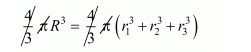# Three solid spheres of radii 3,Question:

Three solid spheres of radii 3, 4 and 5 cm respectively are melted and converted into a single solid sphere. Find the radius of this sphere.

Solution:

Let R be the radius of single solid sphere.

Therefore,

Volume of single solid sphere = volume of all three spheres

$\frac{4}{3} \pi R^{3}=\frac{4}{3} \pi r_{1}^{3}+\frac{4}{3} \pi r_{2}^{3}+\frac{4}{3} \pi r_{3}^{3}$$R^{3}=\left(3^{3}+4^{3}+5^{3}\right)$

$R^{3}=27+64+125$

$R^{3}=216$

$R=\sqrt{216}$

$=6$

$R=6$

Hence, the radius of single solid sphere = 6 cm.